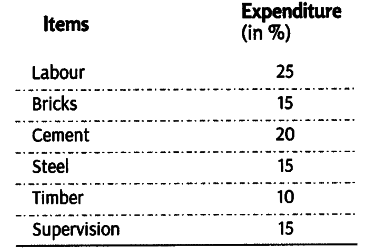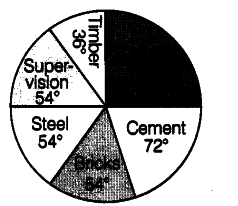# Construct a pie diagram

Construct a pie diagram to represent the cost of construction of a house in Delhi.For constructing a pie diagram, it is necessary to convert the percentage into angles of different degree. This conversion is shown belowA pie diagram to show cost of construction of a house in Delhi is given below